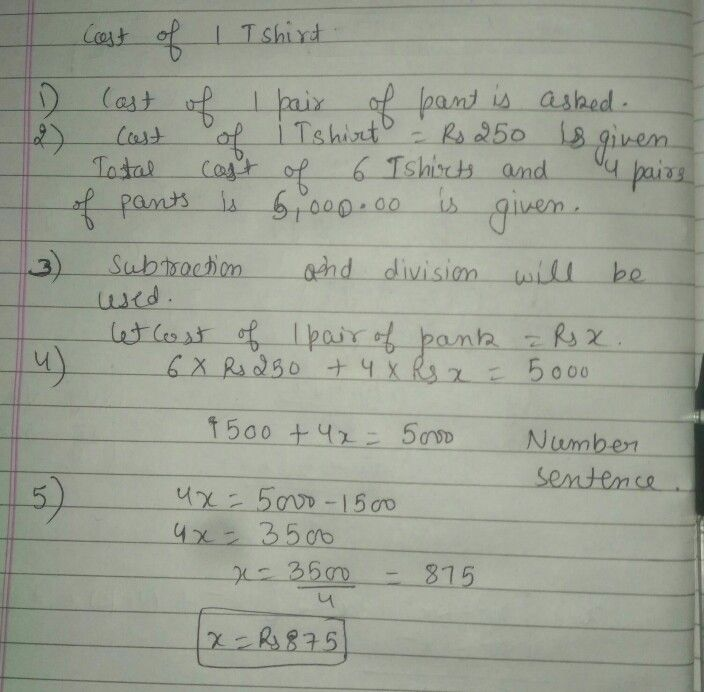Symbol
ProblemLearning Task $1:$ Read and analyze the given $problcm$ Answer the given questions in your notebook. Salima bought $6T$ $T-shi\pi s$ and $4$ pairs of pants for $P5oo0.00$ If the $T$ shirts cost $P250.o0$ cach, find the cost of each pair of pants. $1.$ What is asked? $4$ What is the number sentence? $2$ What are the given? $5$ What is the answer to the problem $3.y$ What operation will be used? $\square$